A variance-covariance matrix expresses linear relationships between variables. Given the covariances between variables, did you know that you can write down an invertible linear transformation that "uncorrelates" the variables? Conversely, you can transform a set of uncorrelated variables into variables with given covariances. The transformation that works this magic is called the Cholesky transformation; it is represented by a matrix that is the "square root" of the covariance matrix.

### The Square Root Matrix

Given a covariance matrix, Σ, it can be factored uniquely into a product Σ=UTU, where U is an upper triangular matrix with positive diagonal entries and the superscript denotes matrix transpose. The matrix U is the Cholesky (or "square root") matrix. Some people (including me) prefer to work with lower triangular matrices. If you define L=UT, then Σ=LLT. This is the form of the Cholesky decomposition that is given in Golub and Van Loan (1996, p. 143). Golub and Van Loan provide a proof of the Cholesky decomposition, as well as various ways to compute it.

Geometrically, the Cholesky matrix transforms uncorrelated variables into variables whose variances and covariances are given by Σ. In particular, if you generate p standard normal variates, the Cholesky transformation maps the variables into variables for the multivariate normal distribution with covariance matrix Σ and centered at the origin (denoted MVN(0, Σ)).

### The Cholesky Transformation: The Simple Case

Let's see how the Cholesky transofrmation works in a very simple situation. Suppose that you want to generate multivariate normal data that are uncorrelated, but have non-unit variance. The covariance matrix for this situation is the diagonal matrix of variances: Σ = diag(σ21,...,σ2p). The square root of Σ is the diagonal matrix D that consists of the standard deviations: Σ = DTD where D = diag(σ1,...,σp).

Geometrically, the D matrix scales each coordinate direction independently of the other directions. This is shown in the following image. The X axis is scaled by a factor of 3, whereas the Y axis is unchanged (scale factor of 1). The transformation D is diag(3,1), which corresponds to a covariance matrix of diag(9,1). If you think of the circles in the top image as being probability contours for the multivariate distribution MVN(0, I), then the bottom shows the corresponding probability ellipses for the distribution MVN(0, D).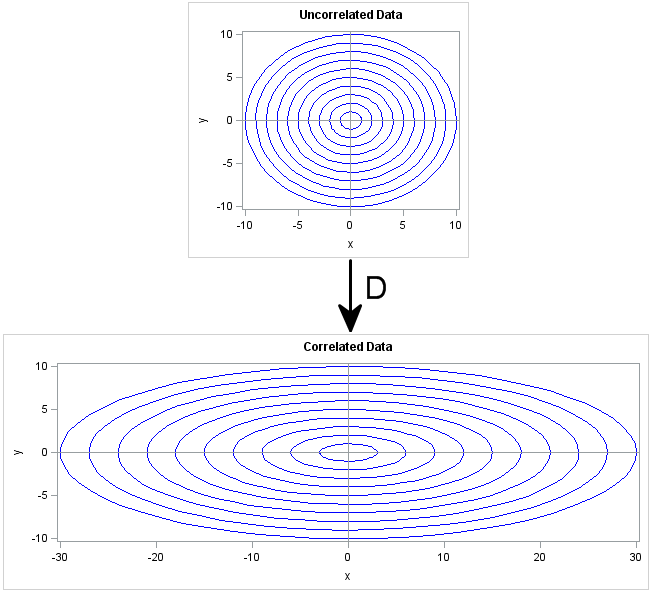### The General Cholesky Transformation Correlates Variables

In the general case, a covariance matrix contains off-diagonal elements. The geometry of the Cholesky transformation is similar to the "pure scaling" case shown previously, but the transformation also rotates and shears the top image.

Computing a Cholesky matrix for a general covariance matrix is not as simple as for a diagonal covariance matrix. However, you can use ROOT function in SAS/IML software to compute the Cholesky matrix. Given any covariance matrix, the ROOT function returns a matrix U such that the product UTU equals the covariance matrix and U is an upper triangular matrix with positive diagonal entries. The following statements compute a Cholesky matrix in PROC IML:

```proc iml; Sigma = {9 1, 1 1}; U = root(Sigma); print U (U`*U)[label="Sigma=U`*U"];```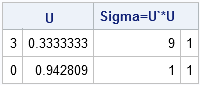You can use the Cholesky matrix to create correlations among random variables. For example, suppose that X and Y are independent standard normal variables. The matrix U (or its transpose, L=UT) can be used to create new variables Z and W such that the covariance of Z and W equals Σ. The following SAS/IML statements generate X and Y as rows of the matrix xy. That is, each column is a point (x,y). (Usually the variables form the columns, but transposing xy makes the linear algebra easier.)The statements then map each (x,y) point to a new point, (z,w), and compute the sample covariance of the Z and W variables. As promised, the sample covariance is close to Σ, the covariance of the underlying population.

```/* generate x,y ~ N(0,1), corr(x,y)=0 */ xy = j(2, 1000); call randseed(12345); call randgen(xy, "Normal"); /* each col is indep N(0,1) */   L = U`; zw = L * xy; /* apply Cholesky transformation to induce correlation */ cov = cov(zw`); /* check covariance of transformed variables */ print cov;```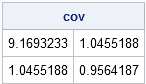The following graph shows the geometry of the transformation in terms of the data and in terms of probability ellipses. The top graph is a scatter plot of the X and Y variables. Notice that they are uncorrelated and that the probability ellipses are circles. The bottom graph is a scatter plot of the Z and W variables. Notice that they are correlated and the probability contours are ellipses that are tilted with respect to the coordinate axes. The bottom graph is the transformation under L of points and circles in the top graph.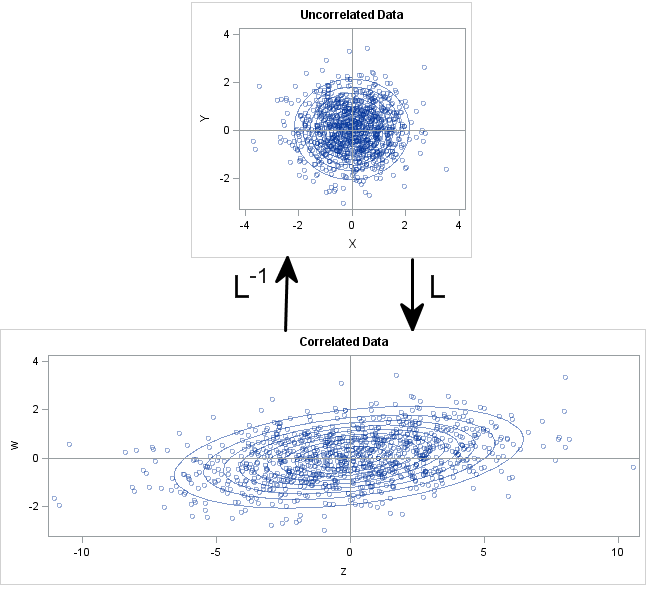### The Inverse Cholesky Transformation Uncorrelates Variables

You might wonder: Can you go the other way? That is, if you start with correlated variables, can you apply a linear transformation such that the transformed variables are uncorrelated? Yes, and it's easy to guess the transformation that works: it is the inverse of the Cholesky transformation!

Suppose that you generate multivariate normal data from MVN(0,Σ). You can "uncorrelate" the data by transforming the data according to L-1. In SAS/IML software, you might be tempted to use the INV function to compute an explicit matrix inverse, but as I have written, an alternative is to use the SOLVE function, which is more efficient than computing an explicit inverse matrix. However, since L is a lower triangular matrix, there is an even more efficient way to solve the linear system: use the TRISOLV function, as shown in the following statements:

```/* Start with correlated data. Apply inverse of L. */ zw = T( RandNormal(1000, {0, 0}, Sigma) ); xy = trisolv(4, L, zw); /* more efficient than inv(L)*zw or solve(L, zw) */   /* did we succeed? Compute covariance of transformed data */ cov = cov(xy`); print cov;```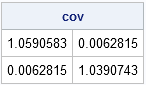Success! The covariance matrix is essentially the identity matrix. The inverse Cholesky transformation "uncorrelates" the variables.

The TRISOLV function, which uses back-substitution to solve the linear system, is extremely fast. Anytime you are trying to solve a linear system that involves a covariance matrix, you should try to solve the system by computing the Cholesky factor of the covariance matrix, followed by back-substitution.

In summary, you can use the Cholesky factor of a covariance matrix in several ways:

• To generate multivariate normal data with a given covariance structure from uncorrelated normal variables.
• To remove the correlations between variables. This task requires using the inverse Cholesky transformation.
• To quickly solve linear systems that involve a covariance matrix.

ShareDistinguished Researcher in Computational Statistics

Rick Wicklin, PhD, is a distinguished researcher in computational statistics at SAS and is a principal developer of SAS/IML software. His areas of expertise include computational statistics, simulation, statistical graphics, and modern methods in statistical data analysis. Rick is author of the books Statistical Programming with SAS/IML Software and Simulating Data with SAS.

1.Does it work for binary data or only for continuous data ?

•Hi Thanh, good to hear from you. The short answer is "only continuous." A longer answer is that often people generate correlated binary data by first generating correlated normal data, and then setting B_i = (X_i > 0). So this approach is INDIRECTLY related to generating binary data. For example, see the paper by Leisch, Weingessel, and Hornik: http://epub2.wu.ac.at/dyn/virlib/wp/showentry?ID=epub-wu-01_221

2.Does this only work in SAS/IML, or is there a way to implement it via Base/STAT/Enterprise Guide/etc?

•It "works" in any product in which you can compute a Cholesky decomposition, but I don't know how to compute that decomposition in Base SAS or SAS/STAT software. (Enterprise Guide can call any product that you have installed, including SAS/IML.)

There are other similar techniques, although their geometry is not as simple. For example, in the book SAS for Monte Carlo Studies: A Guide for Quantitative Researchers, by Fan, Felsovalyi, Sivo, and Keenan, they propose using PROC FACTOR to compute a "factor pattern matrix" from a covariance matrix. The factor pattern matrix is not lower triangular, but it also maps uncorrelated variables into correlated variables. With some work, you can get the DATA step to do the matrix multiplication, but it isn't pretty. If you are planning to do serious simulation studies, I strongly encourage you to consider SAS/IML.

•Thanks for the reply. By "works" I meant the specific code you used to implement it, not the general concept for the Cholesky decomp. Or, more generally, the implementation of its calculation from within SAS... I haven't been able to figure out a way to do it in Base or STAT, which is why I asked.

•The code I posted requires SAS/IML software.

3.Is there a way to ensure a matrix is positive definite for the root function? Or to transform the matrix to positive definite?

Thanks

•Thanks.
That paper's algorithm worked great on your 3x3 example from your link but any advice on how to improve the speed it takes to run on a 184x184 matrix? Currently, it seems to be taking a really long time (i.e. longer than over night if at all).

•You either made a mistake or you are doing something inefficient. The most expensive part of Higham's algorithm is computing the eigenvalues, and that happens in a fraction of a second for a 184x184 matrix (See this plot of the time it takes to compute eigenvalues.) For a matrix of that size, the computation should take only a few seconds.

•I think the time is in convergence. calculating eigenvalues is not the problem. it's running the algorithm until my matrix is positive definite and I can run the root(matrix) function.
I'll take a look again.

Thanks

4.So, since Cholesky factorization of a covariance matrix seems to be closely related to its off-axis rotation/shear, is there some clever and fast way to compute the eigenvectors of Sigma given its LL^T decomposition? Thanks!

•I don't think so. The Cholesky and the spectral (eigenvalue) decompositions are related, but I don't think you can use the Cholesky to obtain the spectral decomposition. On the other hand, you CAN get the Cholesky if you have the spectral. Let A = UDU' where D is the diagonal matrix of eigenvalues and U is the matrix of eigenvectors. Then perform a QR decomposition of sqrt(D)U' = QR where R is upper triangular. Then R`R is a Cholesky decomposition of A.

5.suppose that you get the standard errors for the Cholesky elements of a covariance matrix. I imagine that you can also then get the standard errors for the covariance matrix, but I'm not sure exactly how to do this. any suggestions? it seems like it would involve the delta method.

6.Can I use this method to calculate the Mahalanobis distance between two multivariate normal distributions with different means and different covariance matrices?

•You can use the MD to find the distance from an observation to the center of a cloud of points from a MVN distribution. If there are several clouds (representing different groups or classes), you can use the distance to each group to help classify the observation into one of those groups. See my article on "how to compute the Mahalanobis distance in SAS."

•I need the distance between the means of two MVN distributions, not the distance from an observation to the center of a cloud of points. I know that MD in this case is asymmetric. And as I need a metric, If I use Cholesky transformation to uncorrelate both distributions, can I use Euclidean distance for this goal? If not, could you please recommend a method for that?

•What you propose doesn't make sense to me. Sometimes the pooled covariance is used to compute the distance between centers. For a discussion, links, and bibliography, see the overview of the DISCRIM procedure in the SAS/STAT documentation.

7.hi
is there any way to do correlated variables uncorrelated by Cholesky Transformation in R pack>

8.Thank for article. I have question that does the new generated (correlated) data have same mean and variance as the original before transformation?

•No. This algorithm begins with the standard uncorrelated distribution (zero mean, identity covariance matrix) and ends up with distribution that has zero mean and covariance matrix Sigma. You can add a vector mu = (mu_,...,mu_n) to translate the correlated data so that it has nonzero mean. In the SAS/IML language, the RANDNORMAL function does both of these actions (correlate and translate).

9.I understand that cholesky correlation could generate more and additional data, at this moment I have collected 190 datas from responden, I am running a regressen model using SPSS stepwise. unfortunately the output show un significant among the independent variable. it is againt the theories from the literature review. The regressen equation is Ydependent variable = @ + &Independent Variable + \$ Independent Variable + # Independent Variable. Therefor I am seeking assistant from you to get a formula of order to be run in the syntax, in order to generate more data to 700 respondent. Please respon.

•If the data are not significant predictors of the response, then that is what the data show. You can either gather more data (expensive) or revise your model. Or you might be using the software incorrectly, in which case you should consult an SPSS support community.

If you simulate data and then define the response to be "Model + error," the simulated data will fit the model exactly (because you constructed Y from the model)! It would be unethical to use simulated data to augment real data in order to attempt to generate significant results.

10.This is easier to understand than wiki. Good work!:)

11.Hi,
I have a question, and it would be appreciated if could answer.
To generate correlated random variables, there are two methods ( in fact I know two methods):
Given covariance matrix KY and zero mean unit variance random variables X, we can find A such that, Y=AX. The problem is finding A. To find A we can use two methods. More precisely, A can be computed by Choleskey decompostion, or it can be computed by eigenvectors.
My question is which method (Choleskey , or eigenvectors) is more efficient, and what the advantage or disadvantage of each method is.
Best

•Cholesky is more efficient, and that is the primary advantage. Also, the Cholesky matrix is triangular, which means that Y1 depends on X1, Y2 depends on X1 and X2, and so on. The advantage of an eigenvector decomposition is that the A matrix is the product of an orthogonal, matrix (the eigenvectors) and a diagonal matrix (the square root of the eigenvalues). For the eigen decomp, Y1 depends on X1, X2, ..., Xn, and the same is true for Y2, Y3, etc. However, the eigen decomp has a nice geometry in terms of variance, since the eigen decomp is equivalent to a PCA decomposition.

12.Daniel Nachajon on

Thank you for this clear explanation. I am trying to convince myself that the Cholesky and/or spectral decomposition will preserve the marginals. Also this method is usually described in the context of iid normals but this is not necessary is it? I know that more computational techniques like Iman Conover will do this and are distribution agnostic.

•If the marginal distributions are nonnormal, there is no reason to expect their shape to be preserved by a Cholesky transformation. You can easily modify the example in this post to simulate uniform variates on (0,1). The Cholesky transformation is a triangular matrix, so the first transformed variable (z1) is uniformly distributed, but the second (z2) is a linear combination of uniforms, which is not itself uniformly distributed.

13.Thanks for the great article.
I'm trying to implement this for discrete complex random variables and by using Matlab. I think I've done it but I don't get the expected identity covariance matrix. could you point me to additional help?

•Discrete variables are tricky. See Chapter 9 of Simulating Data with SAS, which discusses how to generate correlated multivariate binary variales and correlated multivarate ordinal variables. The chapter provides references to the literature as well as SAS/IML programs that should readily translate into MATLAB.

14.Petter Asiling on

Hi Rick, this is a nice article. Could you introduce some other methods to implement the transformation between uncorrelated variables to correlated variables? I mean Cholesky is an efficient way to do this, but I am wondering whether there is another way more convenient than it. Now I have a big-size original sample matrix of several correlated variables and I do separated sampling for every single variable (by using Latin hypercube sampling perhaps). Thus I can get a sample matrix (called A) in which the samples are not correlated. I know I can permute those samples subjected to Σ by using Cholesky. Is that the only way to make A get the correlation?

•The scenario in this article assumes that the data are a random sample from a multivariate distribution. LHS is for designed experiments. LHS requires that you can independently control/vary the inputs. You can't always use LHS when the inputs are correlated. For example, if the explanatory variables are age, weight, and BMI, you might not have any patients in your study who have a low age, high weight, and low BMI. If my answer is not clear, you might try posting your question to the "Cross Validated" discussion forum.

15.Dear Professor Rick,

many congratulations.
After running the proc simnormal. I found negative values of simulated variables.I had input data for 4 variables all positive. It is true that correlations is ngative among two of them?
How do I obtain only positive simulated values.
regards

d.bag

•Think about what you are doing. You are specifying that the data are multivariate normal with a given mean vector and covariance matrix. It is easy to see how your situation occurs, even in univariate data. Suppose you have the data {1,1,4}. The mean is 2 and the std dev is sqrt(3)=1.73. If you simulate from the N(2, 1.73) distribution, you will quickly encounter negative values, even though your original data are positive. The underlying reason is that a normal distribution is not a good fit for these data.

You have a few choices. (1) Use a different model for your simulation. For example, model the data by lognormal or exponential. (2) Delete the negative values (this assumes a truncated normal model) or use x=max(x,0) to cap the lower values at 0.

16.Hello,

I am trying to simulate the returns of a portfolio composed of 20 variables in excel. I am using the =norm.inv(rand(),mean, standard deviation) function. Before integrating correlations between the variables using Cholesky decomposition, I standardized the data, i.e. (x-mean)/standard deviation. Once the correlation have been integrated, I de-standardized the data, basically reversed the process of standardization, to keep the same mean and standard deviation of each variable. I am wondering if this is correct from the statistical point of view. I am not sure standardization of data is necessary before applying Cholesky decomposition. It would be great to find a reference to some kind of scientific paper. I need this for work. Your help would be much appreciated. Thank you!

•The ideas in this blog post are also presented in Wicklin, R. (2013) Simulating Data with SAS, SAS Institute, pp. 146-150. No, you do not need to standardize the variables.

17.This explanation is super intuitive and amazing!
Eventually I get some concept about covariance matrix and its square-root!
Thank!